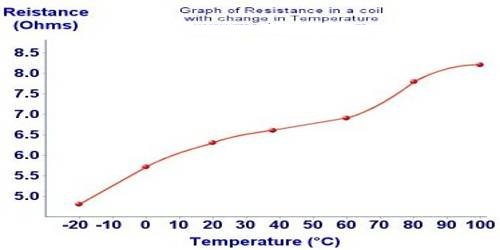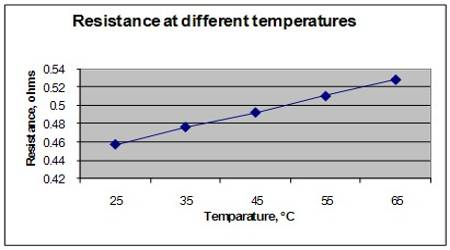# Effect of Temperature on resistance

Effect of Temperature on resistance

If you look at the coil of a heater you will see that as soon as current flows through the coil it becomes hot and bright red. Have you ever for about its reason? It becomes hot due to the resistance of the conductor. When current flows through a conductor it gets hindered by the resistance of the conductor. This property of hindrance is called resistance of the conductor. So it can be said – “The property for which the conductor gives hindrance to the flow of current through it is called resistance.”

Normally, the resistance of the conductor increases if temperature increases and resistance decreases when temperature decreases. But an exception is found in case of carbon. Resistance decreases in carbon when the temperature increases. It is to be remembered that resistance is proportional to temperature. The temperature coefficient of resistance establishes a relation between temperature and resistance. Resistance increases, in case of a conductor, with an increase in temperature and decreases, in case of an insulator, due to increase in temperature.

The resistance of a conductor depends on temperature. Change of resistance with the change of temperature is expressed by the temperature coefficient of resistance. Now we will see what is the reason his thus heat generation. Due to the flow of current in an electric circuit heat is produced which can be explained by electron theory. In a conductor, there are many free electrons. When potential differences are applied between the terminals of a conductor-free electrons move through the intermolecular spaces and are engaged in contains with atoms and molecules. As a result, resistance is produced in the conductor. When temperature increases more thermal energy is acquired by the atoms and molecules and their vibration increases. Consequently, collisions with free electrons are increased. Resistance also increases with it. As a result, the conductor becomes hot.The resistance of a conductor depends on its temperature. Normally, resistance increases as temperature increase, and resistance decreases as temperature decreases. Suppose resistance of a conductor at 00C is R0 and at t0C its resistance is Rt, then

Rt = R0 (1 + αt)

Here, α = constant. It is called the temperature coefficient of resistance.

So, α = (Rt – R0) / R0t

lf R0 = 1, t = 10 then, α = (Rt – R0).

The change of resistance of a conductor having unit resistance that occurs for each Celsius increase of temperature is called temperature coefficient of resistance of that conductor.

Unit of temperature coefficient is (0C)-1 or (K-1).

The temperature coefficient of resistance of aluminum is 3.9 x 10-3 (°C)-1; means that if the temperature of an aluminum conductor having 1Ω resistance is increased by 1°C its resistance increases by 3.9 x 10-3 Ω.